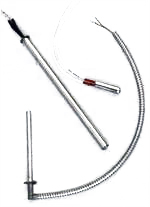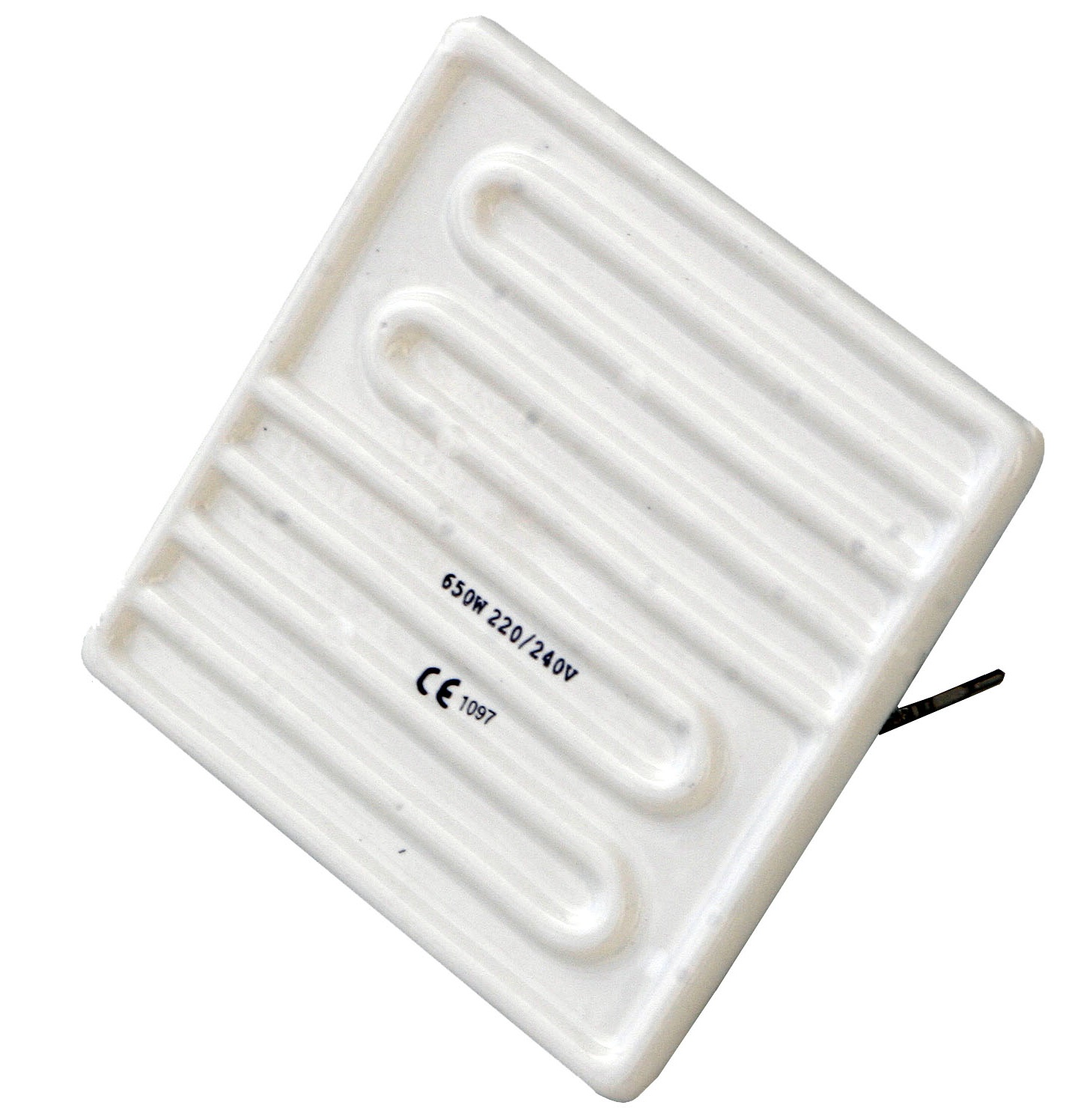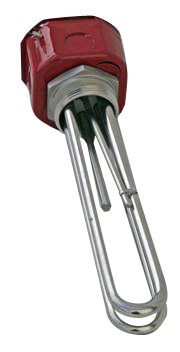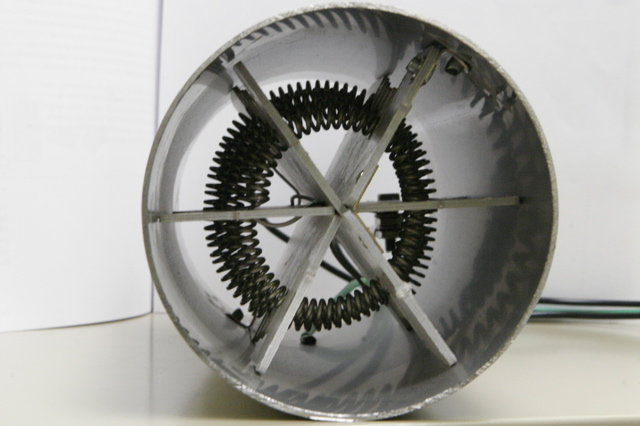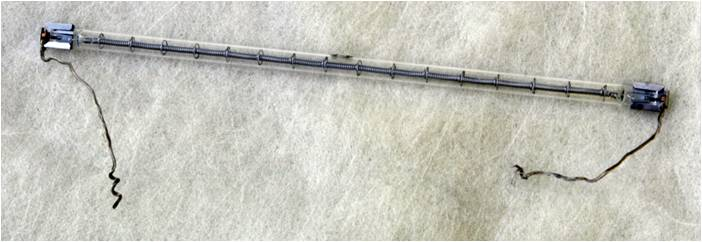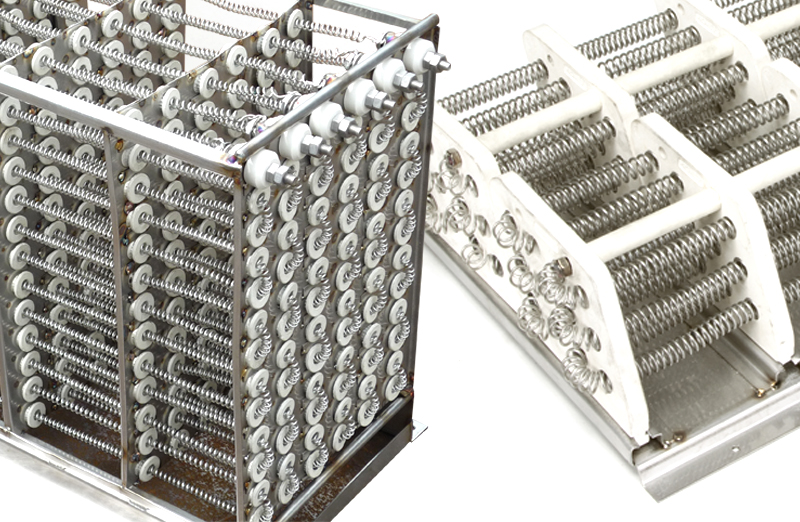Home Basic Industrial Heating Know How Technical Calculations for Industrial Heating Thermal Energy Costs Standard and Norms Technical Papers Credit Application RFQ and Contact Us

# Calculating Wattage for Industrial Heating Applications

To calculate the overall wattage required you need to calculate the wattage for process start-up, process operation and heat loss. (see also: Quick estimates for thermal wattage requirements)
In this first part we focus on the wattage required for process start-up. Process start-up requires quit often the most wattage, because the temperature difference (Delta T) and the heat up time are the biggest factors. Depending on application, (example: heat loss in fluid tank), other factors need to be taken into consideration.

Before you can calculate the needed wattage, you need to know:

1. The type of material or fluid to be heated
2. The mass of the material (in lbs) to be heated
3. The temperature difference (degree Fahrenheit)
4. The desired time to reach set temperature

Here are a couple examples:

Metals:

How many watts are required to heat up 1 gallon (= 231 cubic in) of Steel, Aluminum or Copper in 1 hour based on a temperature difference of 100�F?

• Steel: (66 lbs, spec. heat = .12) = 232 watts
• Al : (23 lbs, spec. heat = .24) = 162 watts
• Cu : (74 lbs, spec. heat = .095) = 206 watts

If the heat up time is decreased to 30 min, then twice the amounts of Watts are required. The efficiency of the heating method (conduction, convection, radiation) as well as the heater type will have a significant impact on power requirement and therefor operating costs. Typical heaters for the above applications are: cartridge heaters, band heaters, strip heaters and tubular heaters.

Water:

To heat up 20 gallons of water from 60 degF to 110 degF (delta T of 50 degress) you'll need 2400 watts. Water is best heated with immersion heaters or circulation heaters, custom heating systems or flexible heaters for wrap around drums or tanks. (example: freezing protection) For most water heating applications we do recommand the use of a mechanical stirring mechanism.

Air:

• kW = ((SCFM x Delta T) / 3000) x 1.2 This formula can be used for "standard air". (standard air is 70 degF @ 14 PSI) SCFM stands for standard cubic feet per minute and Delta T = exit temperature minus inlet temperature in degrees Fahrenheit.
• The first thing to know is how many pounds of air need to be heated. That's the tricky part because it depends on pressure and temperature of the air.
• Example: to heat up 200 scfm by 200 degF, 13.3 kW are needed. If the air is compressed to 50 PSI, the inlet temp is 500 degF, and the temperature needs to be increased to 700 degF, then you'll need 32 kW. For industrial air heating applications, many different type of air heaters can be used. (example: open coil heating elements, tubular heaters, quartz and ceramic air heaters, duct heaters, hot-air tools and blowers)